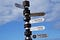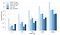# Fast Distance Calculation in PythonPhoto by Bruno on Unsplash

In many machine learning applications, we need to calculate the distance between two points in an Euclidean space. For example, in the k-nearest neighbors (k-NN) algorithm, we need to find the distance between each point in the test dataset to all points in the train dataset and select the train points with smallest distances. In the k-means clustering, the distance between each observation and all cluster centers should be calculated during assignment (association) stage. Therefore, a fast and efficient distance calculation algorithm is highly required.

# Distance in Euclidean Space

The distance between two points in an Euclidean space Rⁿ can be calculated using p-norm operation. Let x =(x1, x2, …,xn) and y =(y1, y2, …,yn) be two points in Euclidean space. Below are the most commonly used distance functions:

1. 1-norm distance (Manhattan distance):

2. 2-norm distance (Euclidean distance):

3. ∞-norm distance (Chebyshev distance):

Basically, you can calculate the distance using any p-norm (p>1) as:

Here, we only focus on the 2-norm distance as it is the most common one, but generalization to other norms can be easily done.

# Python Implementation

The goal is to find all pairwise distances between two sets of points in Rⁿ in Python as fast and efficient as possible. Let X and Y be two matrices with sizes of m × n and k × n, respectively. We would like to calculate the distance matrix D with size of m × k where (i,j)th element of D is the distance between the ith point in X and the jth point in Y. To start, let’s generate our matrices randomly using NumPy library:

Now, we need to create our distance function to calculate all pair-wise distances between all points in X and Y. The easiest way to do this is to create two for-loops; one loop going over each row of X, one loop going over each row of Y and calculate their distance using Euclidean distance equation described above:

Note that on line-5 of the above code, we first need to initialize our distance matrix and then start filling it up with distance values using the two for-loops. This function is clear and easy to understand. However, the major issue is using of for-loops which are very expensive in interpreted languages like Python. As matrix dimensions increase, the complexity and running time of this code grows exponentially which make it impractical. To avoid for-loops in Python, we can take advantages of vector processing in NumPy:

In the above code, we have removed distance matrix D initialization and two expensive for-loops. The subtraction between all data points in X and Y is done using broadcasting and element-wise matrix operation. We will later show the vectorized version is running at least 100x faster than the for-loop version. The only downside is that the vectorized version is not as easy to understand as the for-loop version. Fortunately, there is also a way to optimize the for-loop version:

The trick is to use Numba library. Numba is a just-in-time (JIT) compiler for Python that optimizes NumPy arrays and functions, and loops. All you need to do is to use jit decorator before your NumPy function. The decorator job is to basically compile the decorated function such that it runs without the Python interpreter involvement, leading to much higher performance. Later, in our benchmark test, we will show that the Numba for-loop version is as fast as NumPy vectorized version.

Can we still do better than the NumPy vectorized version? Yes. Interestingly, SciPy library has a distance function which can do better:

You can see our distance function is getting smaller and smaller and actually more efficient. We will show later that cdist function in SciPy is much faster than our NumPy vectorized version. cdist uses a C language function (compiled language) with a Python wrapper. Therefore, when you call cdist in Python, a C function is actually being called which is extremely fast.

Due to emergence of deep learning applications and advancement in hardware technology, GPU processing has become more popular. GPUs operate at lower frequencies than CPUs. However, GPUs have significantly more cores which allow them to process data at much faster rate (by exploiting parallel processing). For example, Intel i7–1160G7 CPU has only 4 cores while Nvidia Tesla V100 has 640 cores. The good news is CuPy library allows us to use GPU accelerated computing in Python. CuPy is written based on NumPy and they have similar syntax:

You can see this function is very similar to our numpy_vectorized function and we just replaced NumPy functions with the corresponding CuPy ones. We also need to change the data type from NumPy to CuPy and vice versa. The only downside of using CuPy is the overhead of transferring data between the CPU and GPU.

# Performance Benchmark

We have introduced 5 different methods to calculate the pairwise distance. The questions is which one is the winner! We have a script that runs these methods for different array sizes and compare their running time. You can download the script here.Running time of different methods versus matrix size [image by author]

The above figure shows the running time in seconds for different methods and matrix sizes. Note that the y-axis is in log-scale to show the difference among methods better. Below is a summary of observations:

1. NumPy for-loop function has the worse running time.
2. NumPy vectorized version can improve the running time significantly and runs 100x faster than the for-loop version.
3. By using Numba (i.e., jit), you can achieve almost the same running time as the NumPy vectorized version. Hence, Numba is highly beneficial if you still prefer to use simple for-loops.
4. SciPy cdist is the clear winner among these methods for all matrix sizes due to its clean/easy interface and C language backbone.
5. CuPy using GPU is as fast as SciPy on large matrix sizes. For small matrix sizes (e.g., 10), CuPy is not beneficial due to the overhead for transferring data between devices (CPU and GPU).

PhD in ECE | Data Scientist | Coding Enthusiast, https://www.linkedin.com/in/reza-vaghefi-b1829516/